American Journal of Aerospace Engineering
Volume 2, Issue 2, April 2015, Pages: 6-10

Relativity and Aeroelasticity Effects on the Supersonic Objects

Arezu Jahanshir

Buein Zahra Technical University, Department of Eng. Physic, Qazvin, Iran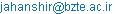Arezu Jahanshir. Relativity and Aeroelasticity Effects on the Supersonic Objects.American Journal of Aerospace Engineering.Vol.2, No. 2, 2015, pp. 6-10. doi: 10.11648/j.ajae.20150202.11

Abstract:Flutter is one of the aerodynamic problems; it mainly occurs on the moving object, especially with wide wings, blade or aerospace vehicles when they cruise at ultra-high speeds. Development and applications of flutter and its related issues in usual speed such as structural design, material section and aerodynamic frame study by many authors like Baurmgart, Jureczko, Guo, Baxevanou and Larsen (see ref. [1-5]). But at ultra-high speeds where the Galilean space and time invariant change to the Lorentz spacetime invariant, the flutter phenomenon will be important to describe the stability of the moving objects at ultra-high speeds. In this limit the torsional stiffness of the wings or the body of the object is very large, so the self-variation causes the instability motion on aerospace-crafts. Therefore, the moving body displacement against the flow field plays an important role in dynamic stability studies. It is the main source of instability in an ultrasonic airplane, which is subjected to aerodynamic forces and velocity of a moving object. Instability and self-oscillation are one of the important reasons of studying the characteristics of an airplane and velocity conditions at the ultra-high speeds, which we can see the relativistic effect of motion, as predicated many years ago by Einstein's theory, i.e. the general theory of relativity. Nowadays, prediction of flutter in the field of aerospace science plays a fundamental role because the aviation safety of ultra-high objects in military and high technology equipment growth day by day. In this article in order to determine the aeroelasticity effects of ultrasonic aerospace-crafts, the theoretical methods based upon physical characteristics of four dimensional spacetime at high velocity (relativity theory) were selected.

Keywords: Lorentz Invariant, Relativity and Aeroelasticity Effects, Supersonic Aerospace-Craft, Relativistic Energy

Contents

1. Introduction

Aeroelasticity or flutter phenomenon in the supersonic aerospace-crafts involved in the study on the interaction between aerodynamic physics and relativity velocity at ultra-high speed. The flexibility of the modern supersonic aerospace-craft’s structures make aeroelastic study an important aspect of modern re-entry equipment and aerospace-craft design and stability verification procedures. The flutter is the major aeroelastic phenomena considered in supersonic moving objects and dynamic aeroelastic instability characterized by sustained self-oscillation of moving object at high speed that arise from interaction between inertial, elastic and aerodynamic forces acting on the moving object with ultra-high speed [6-9]. The values for predication of flutter are taken by the theoretical and experimental results of aerospace-craft, wing, and blade, etc. model. This appears when the aerospace-crafts are subjected to aeroelastic forces at the ultra-high speed. At acute speeds that known as the flutter speed; the supersonic structure sustains oscillations following some initial disturbance. Below this flutter speed the oscillations are damped [11-12], whereas above it any one of the modes becomes negatively unstable oscillations. Aeroelasticity can take various forms involving different pairs of interacting modes and at the high velocity of moving objects. Various approaches to this problem are to describe and determine flutter velocity and moment that will be occurred flutter. As we know in the moving object at ultra-high speed flutter phenomena occurs at a critical speed that is defined as the lowest air velocity at which a moving supersonic object will oscillate with sustained simple harmonic motion [12-14]. Supersonic flight at velocities below and above the flutter speed represents conditions of stable and unstable structural oscillation. It is found most frequently in aerospace-crafts subjected to large wings, tail units and control surfaces and also with ultra-high speed. In engineering studies the forces can interact, causing divergent oscillations for given period differences. The flutter of a supersonic moving object is important example instability that we can study it based on relativistic characteristics of motion. Supersonic-crafts compared to other airplanes have a very thin and wide surface (Fig. 1).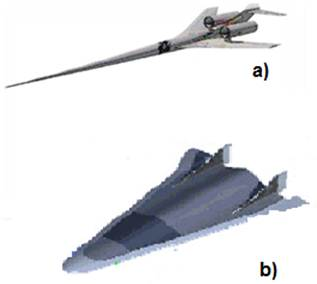Fig. 1. Modern supersonic-crafts: a) The X-54A Low Boom Experimental Vehicle (LBEV), b) HTV3 .

Therefore, supersonic crafts under the influence of aerodynamic forces, especially at ultra-high velocities have specific type of aerodynamic forces that can change its properties and structure constants such as stiffness coefficient and natural frequencies. As a result, the aerospace-craft is faced with strong instabilities that cannot be prevented even by increasing the reliability during the design. This destruction has been created due to a fixed force at ultra-high velocity, and this value of force is created because of a specific relative velocity of flow that presented as flutter phenomenon and the fluid speed destruction presented as flutter speed. Predication the flutter velocity, we can change flight characteristics in order to get the safety of supersonic moving objects. In supersonic-crafts, flutter velocity is considered as the limiting velocity. Limiting velocity is the velocity which must not be reached by an aerospace-craft that depends on the main aerospace-craft’s structure, surface, wings, blade and speed. Therefore, based on physical invariant’s effect of the moving supersonic objects at relativistic spacetime coordinate system, preventing flutter close to fluid velocity can be predicated, if suppose that supersonic-craft move with ultra-high velocity, which is close to the speed of light. Flutter instability is characterized by critical velocity and pulsation in the moving object. To describe flutter we should introduce to physical principles and dynamic model of motion at ultra-high speeds. Mathematical and theoretical model describing aeroelasticity phenomenon is obtained from the Lagrange formalism which consists of the following equation: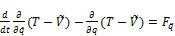(1)

where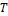is the total kinetic energy,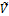is the potential energy,is speed,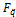is a force, and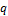is coordinate parameters expressed in terms of generalized coordinates of external forces on the supersonic moving aerospace-crafts. Flutter analysis of supersonic-crafts was often performed using a simple model such as the one shown in Fig. 2. The points P, C, Q, and T, which refers to the reference point, the center of mass, the aerodynamic center, and the three-quarter-of supersonic-crafts. The dimensionless parameters and determine the location of the point C and P, when these parameters are zero, the point lies on the mid of craft length.Fig. 2. Fig. Aeroelastic modeling of supersonic-craft.

In the aerodynamic subjects, the total kinetic energy of the supersonic-craft can be deduced based on the kinetic energy and potential of the mass element, as a result of the flap-wise and torsional velocity and also the flap-wise and torsional stiffness,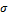andrespectively. Therefore, the total energy for the supersonic- craft is determined as follows :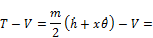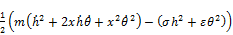(2)

Now, we present the uncoupled frequency and natural frequencies at zero airspeed for detail see :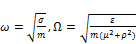(3)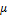is the mass moment of inertia and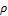is the perpendicular distance between an axis through the center of mass. Here, we consider the dimensionless free stream speed (V) of the air that is sometimes called the reduced velocity: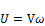(4)

Based on the Lagrange formalism we determine the system of equations and determine the complex roots as functions of V and find smallest value ofto determine flutter speed as follows :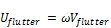(5)

2. Kinetic Energy at Supersonic Speed

In some part of theoretical physics subjects, we have an object that moves with ultra-high speed close to the speed of light, the physical parameters like energy, mass, time will be changed based on the relativistic effect of motion at the ultra-high speed, which described by Einstein and relativistic equations and called ultra-relativistic limit. Description of aeroelasticity at the ultra-high sonic speed should be presented by relativistic theory. In this case, we must present 4D spacetime coordinate system. The theory of 4D spacetime is quite different from the theory of space and time, and also the Euclidean geometry of ordinary three dimensional spaces. The theory of spacetime is relativity theory. It is seen to be a theory of the geometry of the single entity, ‘spacetime’, rather than a theory of space and time [17-21]. The main idea of spacetime is the coordinates of an event have transformation properties analogous to space and time for ordinary three coordinate vectors that involve with a time coordinate. We will consider each event E occurring in spacetime coordinates: (x,y,z,t) in the frame of reference K and (x′,y′,z′,t′) in the new other inertial frame coordinate K′ [15-20]. Relativity theory is the major theory on which modern technology and modern physics are based. This theory is general principles in moving which all specialized theories are required to satisfy and apply to all physical systems that move at ultra-high speeds. The role of relativity appears to be that of specifying the properties of spacetime that all physical processes take place. The principles of relativity are: a) all the laws of physics are the same in every inertial frame of reference. This postulate implies that there is no experiment whether based on the laws of mechanics or the laws of electromagnetism from which it is possible to determine whether a frame of reference is in a state of uniform motion, b) the speed of light is independent of the motion of its source. On the mathematical meaning we can say that the laws of physics are expressed in terms of equations, and the form that these equations take in different reference frames moving with constant velocity with respect to one another can be calculated by using of transformation equations, which require that the transformed equations have exactly the same form in all frames of reference, in other words that the physical laws are the same in all frames of reference. In order to describe the moving object at ultra-high velocity, determination the geometrical properties of spacetime four coordinate, is probably useful. Therefore, we should make use of the constancy of the speed of light and derive the general form that the transformation law must take place. In theoretical field of physics all objects that move with speed near the speed of light have a specific’s motion equation and their dynamical description of motion should be based on the relativistic theory. Thus, hypersonic airplane include in this category. To predicate the occurring moment of flutter in the hypersonic objects we study relativistic combination of spacetime at ultra-high speeds. In the theoretically method, relativistic theory is employed to study the occurring moment of the flutter phenomena. Now, we describe spacetime coordinate relation in relativistic limit [30-31]. As we know, Newtonian mechanics gives an excellent description of motion, but when the object travels very fast, we should use new motion equations, i.e., the Einstein theory that is called special relativity [14, 15]. The effect appears when the speed of moving body or object becomes comparable to the speed of light in the vacuum. In the inertial frames K′ and K coordinate systems that move with relative speed v to each other for simplicity, the direction x is parallel to the direction of motion. Both inertial frames come with Cartesian coordinates: (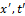) for K′ and (x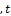) for K, relation between space and time base on Lorentz invariant: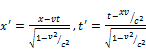(6)

Kinetic energy of motion for the moving object with very small speed compared with the speed of light is Kinetic energy is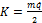i.e. in the Newtonian frame work, but in the 4D framework that involved with velocity value of the moving objects it is completely different. Relativistic kinetic energy equation shows that the energy of an object approaches infinity as the velocity approaches the speed of light. Thus, it is impossible to accelerate an object across this boundary. Relativistic Kinetic energy can be expressed as:(7)

where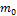is rest mass,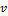is velocity,is speed of light. At an ultra-high sonic speed for the supersonic-crafts, the relativistic kinetic energy should be approximated by equation (7). Thus, the total energy can be partitioned into the energy of the rest mass (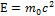) plus the relativistic energy at ultra-high speeds: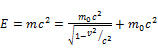(8)

This formula can be blended with the relativistic momentum expression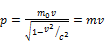(9)

Thus, to give an alternative expression for energy, the combination momentum and energy can be manipulated as follows: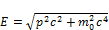(10)

As we see total energy that presented in equation (8) directly depends on the mass and length. Therefore, at the relativistic limit energy, mass, length, time, etc., dependent on the velocity value of the moving object. Thus, the main formula of determination flutter and other aeroelasticity characteristics in the supersonic-crafts involved with relativity effects in spacetime coordinate system.

3. Spacetime Characteristics

In order to understand that how ultra-high velocity effects on spacetime, we try shortly shortly describe relativistic limit of motion and Einstein’s general theory in the four dimensional (4D) spacetime system. The most important achievement of the Lorentz transformation (equation (6)) is relation of time in two coordinate systems. Consider an object placed at rest in a frame of reference K at some point x on the x axis and suppose that this frame is moving with a velocity v relative to some other frame of reference K′. The time interval between two events at K′ and the exact event at K can be determined. Thus, the time interval between events in K is longer than in K′. It means that in the frame of reference K the time is running slow. It appears from K that time is passing more slowly in K′. Hence, after a series of mathematical transformations using relations in (4) we can find time duration in moving spacetime coordinate [17-24]: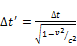(11)

or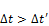(12)

This is the phenomenon of time dilation that we will use to describe how one can predicate events at ultra-high moving spacetime. Now based on relativistic Lorentz invariant we present the length contraction in supersonic moving object. The first of the interesting consequences of the Lorentz transformation is that length no longer has an absolute meaning: the length of an object depends on its motion relative to the frame of reference in which its length is being measured. Let us consider an object moving with a velocity u relative to a frame of reference K′, and lying along the x axis. The object is then stationary relative to a frame of reference K, which is also moving with a velocity v relative to K′. We measure the x coordinates of the two ends of the object at the same time t, as measured by the clocks in K and K′. Turning now to the Lorentz invariant transformation equations (5), we have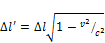(13)

or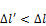(14)

Thus, the length of the moving object as measured in the frame of reference K′ with respect to which the length of moving object is shorter than the length as measured from a frame of reference K relative to which the object is stationary. A moving object will have the maximum length when it is stationary in a frame of reference. The length so-measured, l is known as proper length. This phenomenon is known as the Lorentz contraction. It is not the consequence of some force ‘squeezing’ the object, but it is a really physical phenomenon with observable physical effects. Note, however, that someone who actually looks at this moving object as it passes by will not see a shorter object. If the time that is required for the light from each point on the moving object to reach the observer’s eye is taken into account, the overall effect is that of making the object appear as if it is rotated in spacetime. Now, we suppose that an object has a velocity v relative to a frame K′ and velocity of this object that measure in the frame of reference relative to a frame K moving with a velocity u relative to a frame K′. The moving object has coordinate x at time t in K′ and it has coordinate x at time t in K. On the other words, if the observer in K measures an object moving along the x axis at speed u, then the observer in the K′ coordinate system that moving at speed v in the x direction with respect to K, will measure the object moving with speed u′ where from the Lorentz transformations (5) [20-23]: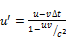(15)

and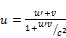(16)

In low speed both u and v are small with respect to the speed of light and we will recover(17)

the intuitive Galilean transformation of velocities. If objects moving with ultrasonic speed βc, we can determine delay time as follows: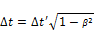(18)

and one may predicate the exact delay time in spacetime coordinate when the flutter will be happened in moving spacetime coordinate.

4. Hypersonic Flutter

Dynamic effects in mechanism of the moving supersonic objects due to aerodynamic characteristics during normal moving or rotation are significant [25-30]. As we know the velocity of moving supersonic-crafts and its stiffness of a structure need to find the various periods at which it will naturally resonate [16,31]. The goal of relativistic analysis in structural mechanics is to determine the time dilation of flutter and natural mode shapes and frequencies of an object or structure during free vibration in second spacetime where supersonic-craft was observed. In order to do relativistic and aeroelastic analysis and ensure the non-occurrence of flutter phenomenon, time dilation in second spacetime coordinate are studied. For hypersonic moving objects using equation (18), we can determine delay time when flutter will be happened in the K′ coordinate system. If objects moving with ultrasonic speed βc, so we can determine delay time.

5. Conclusion

In this study, the relativistic effects of aerodynamic loading at ultra-high velocity were considered. Based on the Lorentz invariant equations the time dilation for flutter was determined. In theoretical method the flutter velocity and natural frequency of the system was studied, and flutter speed related to the coupling of bending, and torsion were defined. We exactly determined that flutter involves with aerodynamic-elastic and velocity at the ultra-high limit. Equations were shown that some critical speed that known as the flutter speed, could be predicated by an observer in the initial frame reference. Therefore, the body displacement against the flow field plays a fundamental role in dynamic solidity analysis. In order to maintain the supersonic object stability in ultrahigh speed, predication’s time in the occurring flutter can be determined in the relativistic limit. The main focus on this study is to merge relativistic equation of motion in mechanical engineering science and determination of predication flutter’s moment.

References

1. A. A. Baumgart, mathematical model for wind turbine blades, J. Sound Vib., 251(1), 1-12, 2002.
2. M.E. Jureczko, M., Pawlak, and A., Mezyk, Optimisation of wind turbine blades, J. Mater. Process. Tech., 167(2), 463-471, 2005.
3. S. Guo, Aeroelastic optimization of an aerobatic aircraft wing structure, Aerospace Sci. Technol., 11(5), 396-404, 2007.
4. C.A. Baxevanou, P.K. Chaviaropoulos, S.G. Voutsinas, and N.S. Vlachos, Evaluation study of a Navier–Stokes CFD aeroelastic model of wind turbine airfoils in classical flutter, J. Wind Eng. Ind. Aerod., 96(8), 1425-1443, 2008.
5. J.W. Larsen, and Nielsen, S.R., Nonlinear parametric instability of wind turbine wings, J. Sound Vib., 299(1), 64-82, 2007.
6. A. Andronov, A. A. Vitt and S. E. Khakin, Theory of Oscillator, Mineola, NY: Dover, 1987.
7. L. Mirovitch, Elements of Vibration analysis, Dover Publications, 2011.
8. L. Raymond et al., Principles of aeroelasticity, Dover Publications, 2002.
9. T.H.G. Megson, Introduction to Aerospace Structural Analysis, Butterworth-Heinemann, 2013.
10. W. C. Hurty and M. F. Rubinstein, Dynamics of Structures, Prantice Hall of India Pvt. Ltd., 1967.
11. H. Babinksy, How do wings work? Phys. Educ. 38, 2003.
12. E. H. Dowell, D. A. Peters, R. H. Scanlan and F. Sisto, A Modern course in Aeroelasticity, III edition, Kluwer Academic Publishers, 1995.
13. a)http://www.uasvision.com/2012/04/04/nasa-tests-supersonic-aircraft-without-boom/, b)http://www.globalsecurity.org/space/systems/x-41-htv-3.htm
14. R. Fitzpatrick, Oscillations and Waves: An Introduction, CRC Press, 2013.
15. P. Scheerbart, The perpetual Motion Machines, Wakefield Press, 2011.
16. A. H. Gasemi, A. Jahanshir, Numerical and analytical study of aero elastic characteristics of wind turbine composite blades, International WIND and Structure Journal, 18(2), 103-116, 2014.
17. H. Goldstein, C. P. Poole and J. L. Safko, Classical Mechanics, 3rd ed., San Francisco: Addison Wesley, 2002.
18. C. Moller, The Theory of Relativity, Oxford, Clarendon Press, 1972.
19. A. Einstein, Relativity: The Special and General Theory (Translation 1920), New York: H. Holt and Company, 1916.
20. S. Carroll, From Eternity to Here: The Quest for the Ultimate Theory of Time, New York: Dutton, 2010.
21. E. J. Saletan, Classical Dynamics: A Contemporary Approach, Cambridge: Cambridge University Press, 1998.
22. S. H. Strogatz et al., Theoretical mechanics: Crowd synchrony on the Millennium Bridge, Nature 438, 43-44, 2005.
23. H. Goldstein, C. P. Poole and J. L. Safko, Classical Mechanics, 3rd ed., Boston: Addison Wesley, 2001.
24. L. D. Landau and E.M. Lifshitz, Mechanics, 3rd ed., Oxford: Elsevier, 1976.
25. A. B. Pippard, The Physics of Vibration, omnibus ed., Cambridge: Cambridge University Press, 1989.
26. R. Altman, Sound theory, Sound practice, Routledge, 1992.
27. A. Jenkins, Self-oscillation, Phy.Reports 525 (2), 167–222. arXiv:1109.6640, 2013.
28. E. H. Dowell, Theoretical and experimental panel flutter study AIAA J., 3(12), 1995.
29. H. J. Pain, The Physics of Vibrations and Waves, 6th ed., Chichester: John Wiley & Sons, 2005.
30. J. Dugundji, Theoretical consideration of Panel flutters at high supersonic Mach No., AIAA J. 4(7), 1966.
31. M. P. Paldoussis, S. Price and E.de Langre, Fluid Structure Interactions: Cross-Flow-Induced Instabilities, Cambridge University Press, 2011.

 Contents 1. 2. 3. 4. 5.
Article ToolsAbstractPDF(471K)